The different devices (or systems) that make up a facility require different amounts of electrical energy. Therefore, estimating the energy consumption that all the devices consume is an essential step every manager or engineer must take. Not only does this information allow facility managers to lower energy usage and reduce monthly electricity bills, but it also allows them to plan for alternative (or backup) energy systems.

## Understanding some electrical terminologies

An electric circuit is formed when a conductive path allows electric charges to move continuously. Electrical appliances and devices harness the movement of these charges in order to do work. And the following terms are useful in understanding how electric circuits work.

• Current: describes the rate at which these charges move in the circuit. It is measured in amperes.
• Voltage: describes the force motivating these charges to flow in the circuit. It is a measure of the potential energy relative between two points in the circuit.
• Resistance: describes the property of a material (or device) that restricts current flow in the circuit. It is measured in Ohms.

Ohm’s law expresses the relationship between voltage, current and resistance in a circuit. This law states that the current flowing in a circuit is directly proportional to the applied potential difference (or voltage) and inversely proportional to the resistance in the circuit, provided the temperature remains constant. It can be expressed mathematically as: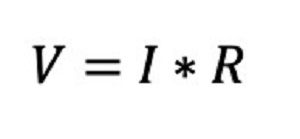Where:

V = voltage

I = current

R = resistance

To better understand how electric circuits work, consider the simple pressurized water system and lightbulb electric circuit in Figure 2. The flow of current through this circuit is analogous to the flow of water in the pressurized water system shown in Figure 2a. However, while the power supply generates a voltage (or electrical pressure) and causes current to flow, the pump creates water pressure in the pipe and causes water to flow. Likewise, the lightbulb provides electric resistance similar to the restriction in the pressurized water system.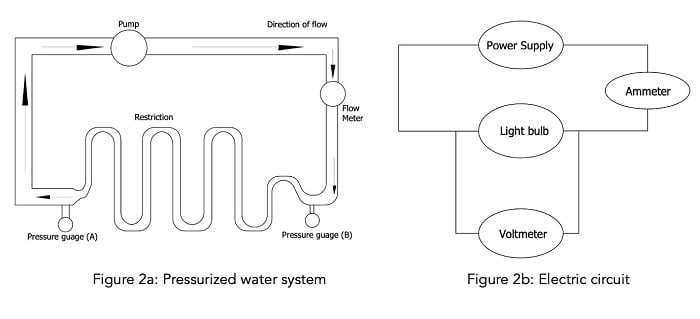Source: Temitayo Oketola

The power generated by this electrical circuit is related to the electric current and voltage, according to the equation below: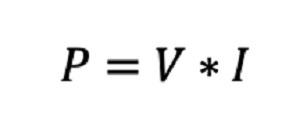Where:

V = voltage (V)

I = current (A)

P = power (Watts)

## Calculating the energy usage

### 1. Calculate the wattage of all the devices

Wattage describes the capacity of an electrical device or the maximum power drawn by the device during operation. An electrical device’s wattage rating is usually printed on its nameplate.

However, since wattage rating is given at extreme operating conditions, the actual amount of power a device consumes under normal operating conditions is much less than what is printed on the nameplate. Therefore, engineers can determine the actual power a device consumes by using wattage monitoring systems.

### 2. Estimate the daily use

After determining the wattage ratings of all devices, engineers must estimate each device's average daily energy consumption. This can be obtained by multiplying the wattage of each device by the number of hours it operates each day, as shown below: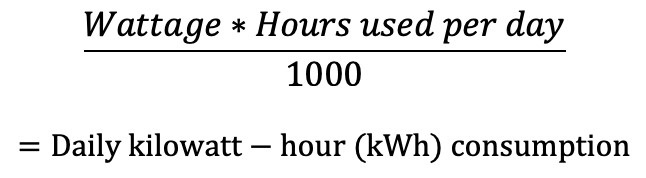For example, consider a fire alarm with a wattage rating of 10 W. Suppose that the fire alarm operates 24 hours per day. In that case, its daily kilowatt-hour energy consumption would be 0.24 kWh. If this alarm system is to be operated every day all year round, its yearly consumption would be 87.8 kWh, as shown below.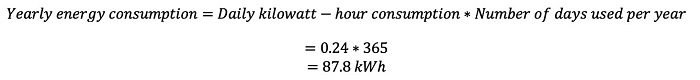Repeat the yearly energy consumption calculation for all the devices and sum the results to obtain a total kWh value.

### 1. Find the annual cost to run the devices

Now that the approximate yearly energy consumption has been determined for all devices, it is possible to estimate how much the energy bill will cost. For instance, if the rate is set at \$0.10/kWh, then the approximate cost per year for the fire alarm system will be obtained as \$8.78, as shown below: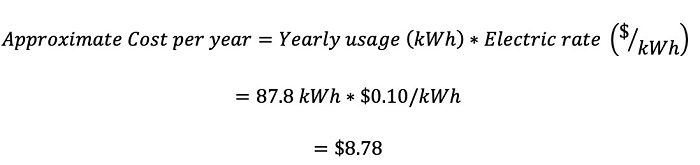## Conclusion

Calculating electricity usage and bills is a good way to ensure that facilities stay on a budget for the month or year. While this article presents helpful tips for calculating energy usage, engineers should note that energy monitoring systems are capable of providing more accurate and detailed data on energy usage.